# Bihar Board Class 9th Maths Solutions Chapter 1 Number Systems Ex 1.3

Bihar Board Class 9th Maths Solutions Chapter 1 Number Systems Ex 1.3 Textbook Questions and Answers.

## BSEB Bihar Board Class 9th Maths Solutions Chapter 1 Number Systems Ex 1.3Question 1.
Write the following in decimal form and say what kind of decimal expansion each has :
(i) $$\frac { 36 }{ 100 }$$
(ii) $$\frac { 1 }{ 11 }$$
(iii) 4$$\frac { 1 }{ 8 }$$
(iv) $$\frac { 2 }{ 11 }$$
(v) $$\frac { 2 }{ 11 }$$
(vi) $$\frac { 329 }{ 400 }$$
Solution:
(i) $$\frac { 36 }{ 100 }$$ = 0.36, terminating.

(ii) By long division, we have∴ $$\frac { 1 }{ 11 }$$ = 0.090909 = 0.$$\overline{09}$$, non-terminating and repeating.

(iii) 4$$\frac { 1 }{ 8 }$$ = $$\frac{4 \times 8+1}{8}$$ = $$\frac { 33 }{ 8 }$$
By long division, we have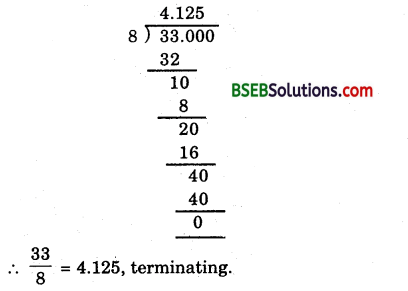(iv) By long division, we have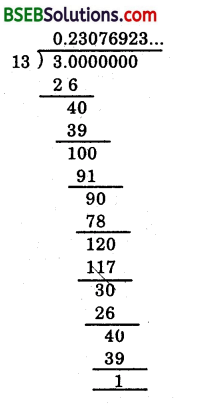∴$$\frac { 3 }{ 11 }$$ = 0.23076923… = $$\overline{0.230769}$$, non-terminating and repeating.

(v) By long division, we have∴ $$\frac { 2 }{ 11 }$$ = 0.181818… = 0.$$\overline{18}$$, non-terminating and repeating.

(vi) By long division, we have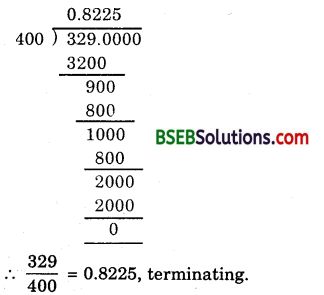Question 2.
You know that $$\frac { 1 }{ 7 }$$ = 0.$$\overline{142857}$$. Can you predict what the decimal expansions of $$\frac { 2 }{ 7 }$$, $$\frac { 3 }{ 7 }$$, $$\frac { 4 }{ 7 }$$, $$\frac { 5 }{ 7 }$$, $$\frac { 6 }{ 7 }$$ are, without actually doing the long division? If so, how?
Solution:
Yes. All of the above will have repeating decimals which are permutations of 1, 4, 2, 8, 5, 7.
For example, here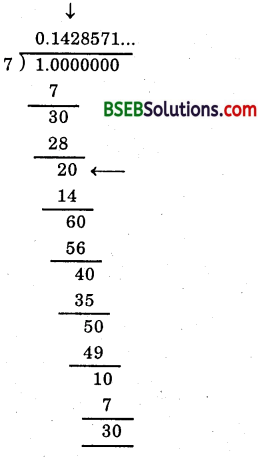To find $$\frac { 2 }{ 7 }$$, locate when the remainder becomes 2 and respective quotient (here it is 2), then write the, new quotient beginning from there (the arrows drawn in the above division) using the repeating digits 1, 4, 2, 8, 5, 7.Question 3.
Express the following in the form $$\frac { p }{ q }$$, where p and q are integers and q ≠ 0.
(i) 0.$$\bar { 6 }$$
(ii) 0.4$$\bar { 7 }$$
(iii) 0.$$\bar { 001 }$$
Solution:
(i) Let x = 0.$$\bar { 6 }$$
Then, x = 0.666 … (1)
Here, we have only one repeating digit. So, we multiply both sides of (1) by 10 to get
10x = 6.66 … (2)
Subtracting (1) from (2), we get
10x – x = (6.66…) – (0.66…)
⇒ 9x = 6 ⇒ x = $$\frac { 2 }{ 3 }$$
Hence, 0.$$\bar { 6 }$$ = $$\frac { 2 }{ 3 }$$

(ii) Let x = 0.4$$\bar { 7 }$$
Clearly, there is just one digit on the right side of the decimal point which is without bar. So, we multiply both sides by x so that only the repeating decimal is left on the right side of the decimal point.
∴ 10x = 4.$$\bar { 7 }$$
⇒ 10x = 4 + 0.$$\bar { 7 }$$
⇒ 10x = 4 + $$\frac { 7 }{ 9 }$$

Rule :

• Write the number without decimal point as numerator.
• Write as many 9’s as there are different repeating digits for the denominator.

For example : 0.$$\bar { 7 }$$ = $$\frac { 7 }{ 9 }$$, 0.$$\overline{35}$$ = $$\frac { 35 }{ 99 }$$ etc.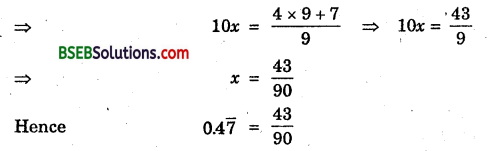Aliter:
Let x = 0.4$$\bar { 7 }$$ = 0.4777 …
∴ 10x = 4.777 …. (1)
and 100x = 47.777 …. (2)
Subtracting (1) from (2), we get
100x – 10x = (47.777…) – (4.777…)
⇒ 90x = 43 ⇒ x = $$\frac { 43 }{ 90 }$$
Hence, 0.4$$\bar { 7 }$$ = $$\frac { 43 }{ 90 }$$

(iii) Let x = 0.$$\overline{001}$$
⇒ x = 0.001001001 … (1)
Here, we have three repeating digits after the decimal point. So, we multiply (1) by 10³ = 1000, we get
1000x = 1.001001 … (2)
Subtracting (1) from (2), we get
1000x – x = (1001001 …) – (0.001001…)
⇒ 999x = 1 ⇒ x = $$\frac { 1 }{ 999 }$$
Hence, 0.$$\overline{001}$$$$\frac { 1 }{ 999 }$$Question 4.
Express 0.99999 …. in the form $$\frac { p }{ q }$$. Are you surprised by your answer? With your teacher and classmates discuss why the answer makes sense.
Solution:
Let x = 0.9999 … (1)
Here, we have only one repeating digit. So, we multiply both sides of (1) by 10 to get
10x = 9.999 …(2)
Subtracting (1) from (2), we get
10x – x = (9.999…) – (0.999…)
⇒ 9x = 9 ⇒ x = 1
Hence, 0.9999… = 1
Since 0.9999… goes on forever. So, there is no gap between 1 and 0.9999… and hence they are equal.

Question 5.
What can the maximum number of digits be in the repeating block of digits in the decimal expansion $$\frac { 1 }{ 17 }$$? Perform the division to check your answer.
Solution:
We have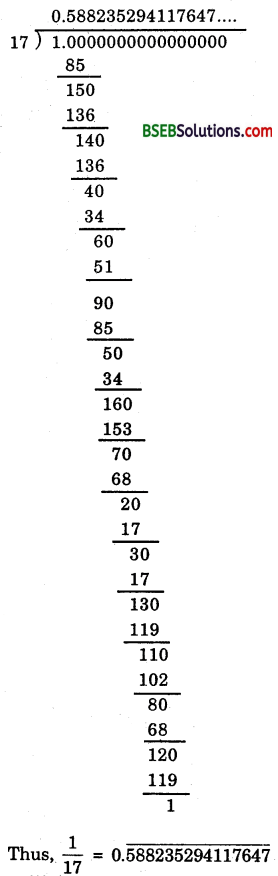∴ The maximum number of digits in the quotient while computing $$\frac { 1 }{ 17 }$$ are 15.Question 6.
Look at several examples of rational numbers in the form $$\frac { p }{ q }$$(q ≠ 0), where p and q are integers with no common factors other than 1 and having terminating decimal representations (expansions). Can you guess what property q must satisfy?
Solution:
Consider several rational numbers in the form $$\frac { p }{ q }$$ (q ≠ 0), where p and q are integers with common factors other than 1 and having terminating decimal representation.
Let the various such rational numbers be $$\frac { 1 }{ 2 }$$, $$\frac { 1 }{ 4 }$$, $$\frac { 7 }{ 8 }$$, $$\frac { 37 }{ 25 }$$, $$\frac { 8 }{ 125 }$$, $$\frac { 17 }{ 20 }$$, $$\frac { 31 }{ 16 }$$ etc.
In all cases, we think of the natural number which when multipled by their respective denominators gives 10 or a power of 10.We have seen that those rational numbers whose denominators when multiplied by a suitable integer produce a power of 10 are expressible in the finite decimal form. But this can always be done only when the denominator of the given rational number has either 2 or 5 or both of them as the only prime factors. Thus, we obtain the following property :
If the denominator of a rational number in standard form has no prime factors other than 2 or 5, then and only then it can be represented as a terminating decimal.

Question 7.
Write three numbers whose decimal expansions are non-terminating non-recurring.
Solution:
Three numbers whose decimal representations are non-terminating and non-repeating are $$\sqrt{2}$$, $$\sqrt{3}$$ and $$\sqrt{5}$$ or we can say 0.100100010001…, 0.20200200020002… and .003000000003…

Question 8.
Find three different irrational numbers between the rational numbers $$\frac { 5 }{ 7 }$$ and $$\frac { 9 }{ 11 }$$.
Solution:
We haveThus, three different irrational numbers between $$\frac { 5 }{ 7 }$$ and $$\frac { 9 }{ 11 }$$ are
0. 75075007500075000075…, 0.767076700767000… and 0.80800800080000…Question 9.
Classify the following numbers as rational or irrational :
(i) $$\sqrt{23}$$
(ii) $$\sqrt{225}$$
(iii) 0.3796
(iv) 7.478498 …
(v) 1.101001000100001…
Solution:
(i) $$\sqrt{23}$$ is an irrational number as 23 is not a perfect square.
(ii)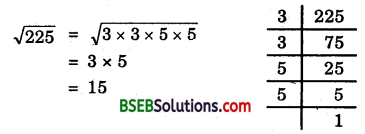Thus, 15 is a rational number.
(iii) 0.3796 is a rational number as it is terminating decimal.
(iv) 7.478478… is non – terminating but repeating, so, it is a rational number.
(v) 1.101001000100001… is non-terminating and non-repeating so, it is an irrational number.